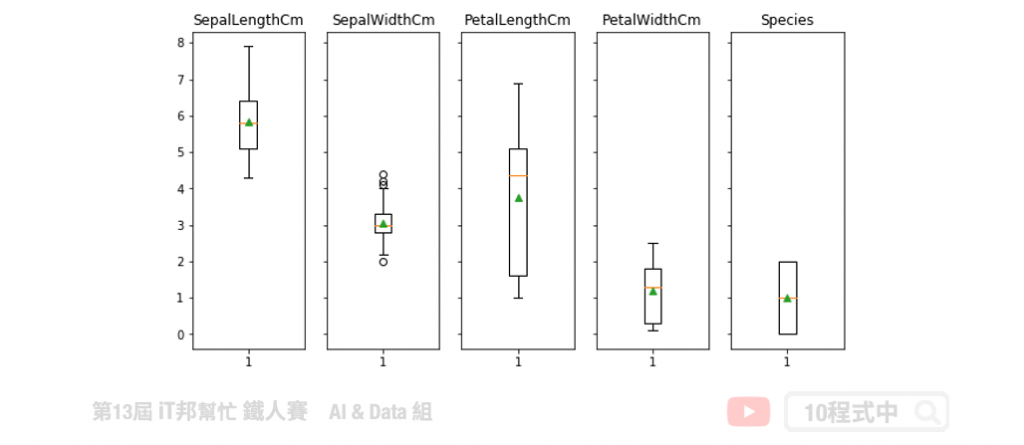DAY 3
1
AI & Data

# 你真了解資料嗎？試試看視覺化分析吧！

## 今日學習目標

• 探索式分析 (EDA)
• 聊聊何謂 EDA，為何要做數據分析?
• 撰寫第一支 EDA 程式
• 透過鳶尾花 (iris) 資料集，來查看資料的分佈狀態

## EDA 必要的套件

• 資料處理 – Pandas, Numpy
• Pandas：Python 表格資料處理的重要工具
• Numpy：針對多維陣列的平行運算進行優化的強大函式庫
• 繪圖相關 – Matplotlib, Seaborn
• Matplotlib：Python 最常被使用到的繪圖套件
• Seaborn：以 matplotlib 為底層的高階繪圖套件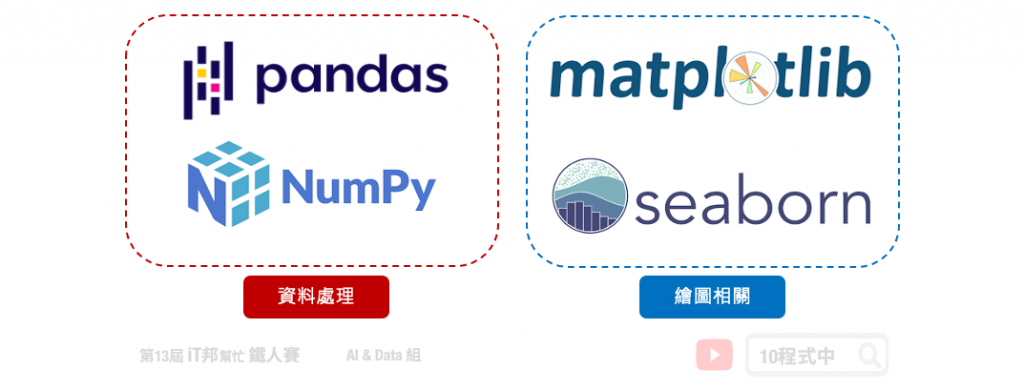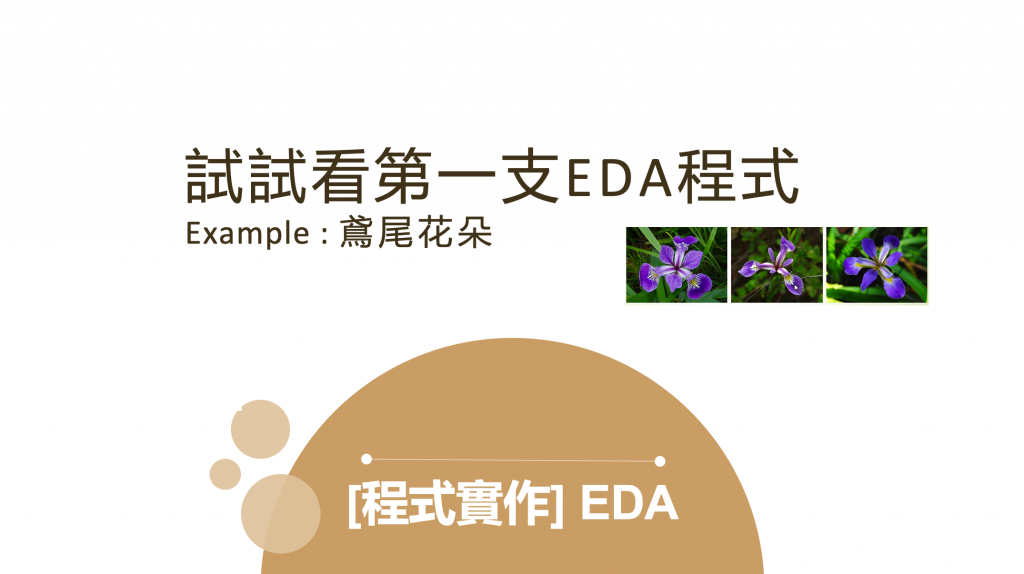## 鳶尾花朵資料集一覽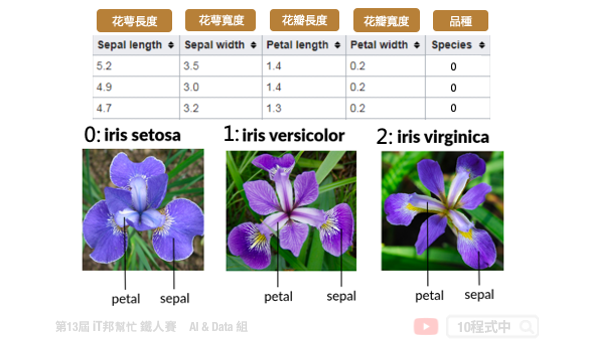## 載入必要套件

`pandas`、高階大量的維度陣列與矩陣運算的 `numpy`、處理資料視覺化的繪圖庫 `matplotlib``seaborn`。最後一個是資料集來源，此系列範例我們採用 Sklearn 所提供的鳶尾花分類的資料集。

``````import pandas as pd
import numpy as np
import matplotlib.pyplot as plt
import seaborn as sns
``````

## Sklearn Toy datasets

Sklearn 套件中提供了七個快速入門的 Toy datasets 很推薦初學者可以載入來玩玩看，並且練習做資料探索與建模。每一個資料集呼叫的方法非常簡單。以鳶尾花朵資料集為例，我們可以透過 API 取得輸入與輸出。

``````from sklearn.datasets import load_iris

# 輸入特徵
X = iris.data
# 輸出特徵
y = iris.target
``````

Sklearn 提供了許多 API 方法可以呼叫：

• data: 取得輸入特徵
• target: 取得輸出特徵
• feature_names: 取得輸入特徵的名稱
• target_names: 取得輸出的類別標籤(分類資料集)
• DESCR: 資料集詳細描述

## 載入資料集

``````iris = load_iris()
df_data = pd.DataFrame(data= np.c_[iris['data'], iris['target']],
columns= ['SepalLengthCm','SepalWidthCm','PetalLengthCm','PetalWidthCm','Species'])
df_data
``````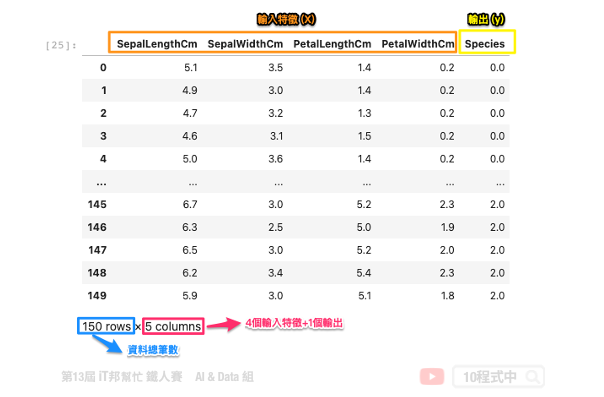## 直方圖

``````#直方圖 histograms
df_data.hist(alpha=0.6,layout=(3,3), figsize=(12, 8), bins=10)
plt.tight_layout()
plt.show()
``````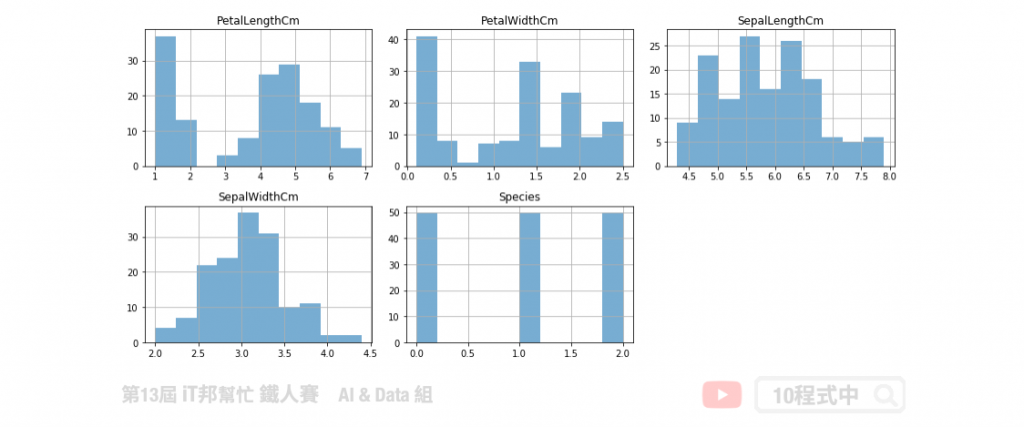``````fig, axes = plt.subplots(nrows=1,ncols=4)
fig.set_size_inches(15, 4)
sns.histplot(df_data["SepalLengthCm"][:],ax=axes, kde=True)
sns.histplot(df_data["SepalWidthCm"][:],ax=axes, kde=True)
sns.histplot(df_data["PetalLengthCm"][:],ax=axes, kde=True)
sns.histplot(df_data["PetalWidthCm"][:],ax=axes, kde=True)
``````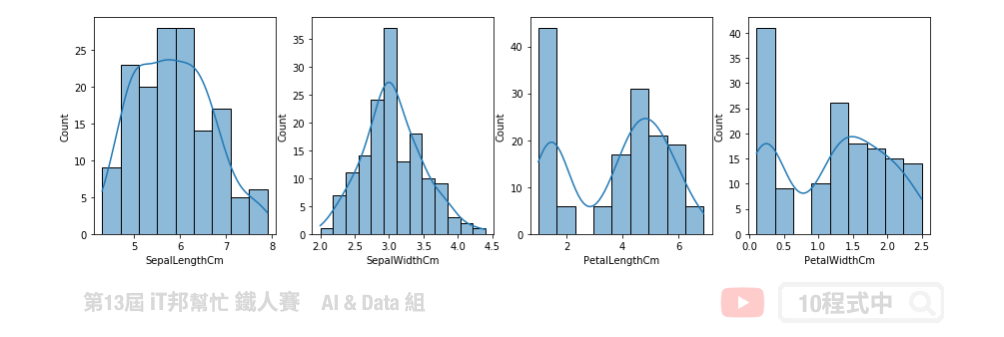## 核密度估計

``````from pandas.plotting import scatter_matrix
scatter_matrix( df_data,figsize=(10, 10),color='b',diagonal='kde')
``````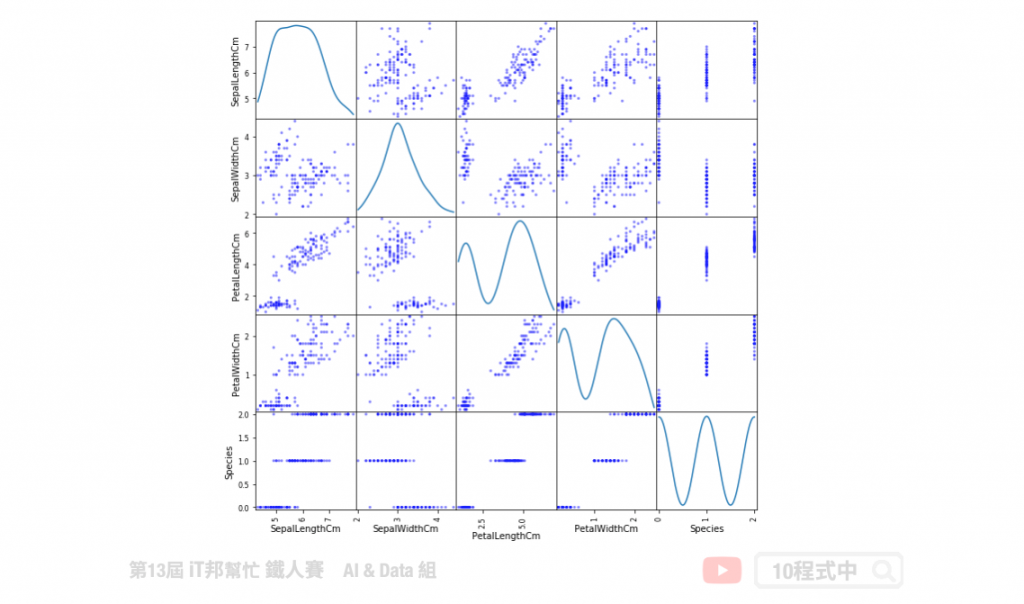``````sns.pairplot(df_data, hue="Species", height=2, diag_kind="kde")
``````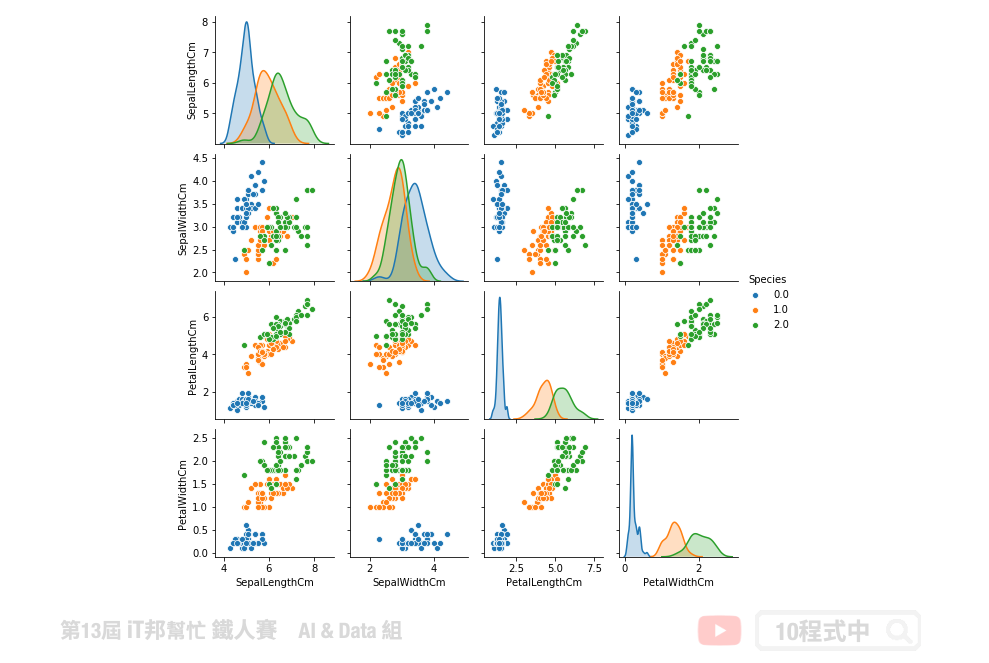## 關聯分析

``````# correlation 計算
corr = df_data[['SepalLengthCm','SepalWidthCm','PetalLengthCm','PetalWidthCm','Species']].corr()
plt.figure(figsize=(8,8))
sns.heatmap(corr, square=True, annot=True, cmap="RdBu_r")
``````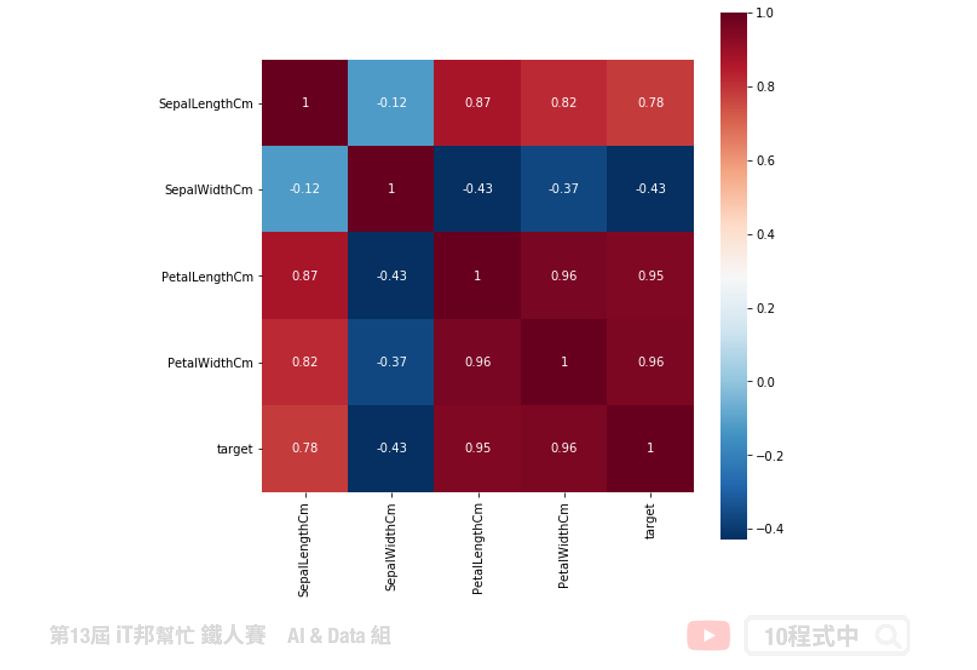## 散佈圖

``````sns.lmplot("SepalLengthCm", "SepalWidthCm", hue='Species', data=df_data, fit_reg=False, legend=False)
plt.legend(title='Species', loc='upper right', labels=['Iris-Setosa', 'Iris-Versicolour', 'Iris-Virginica'])
``````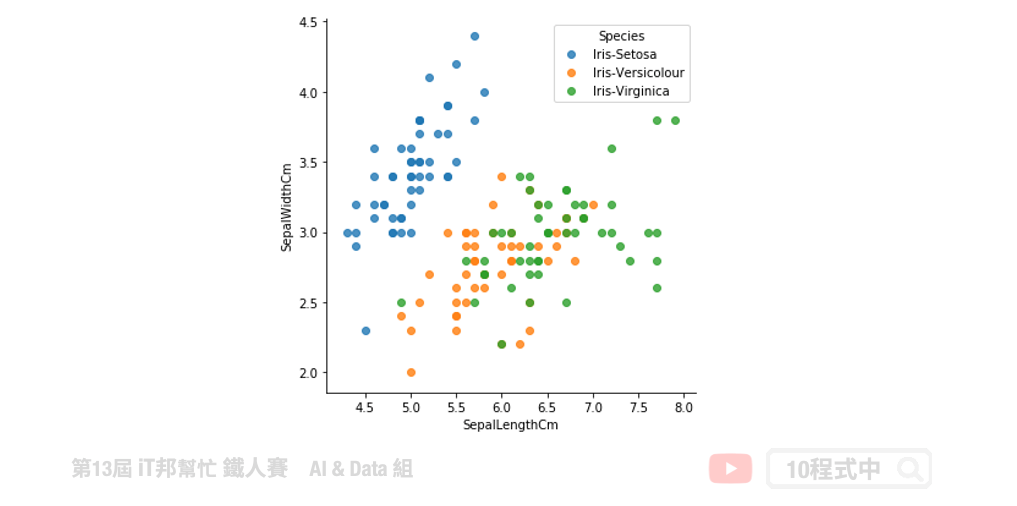## 箱形圖